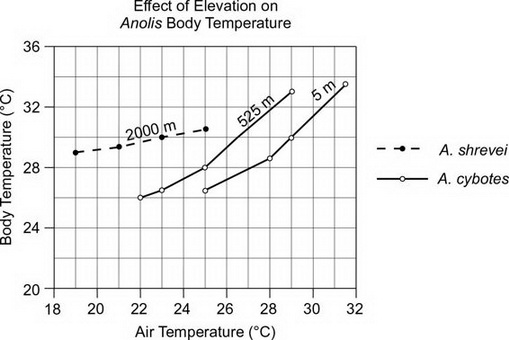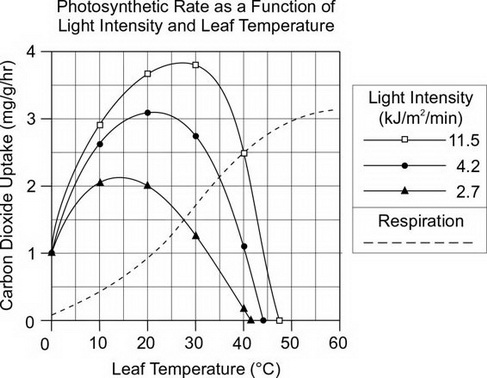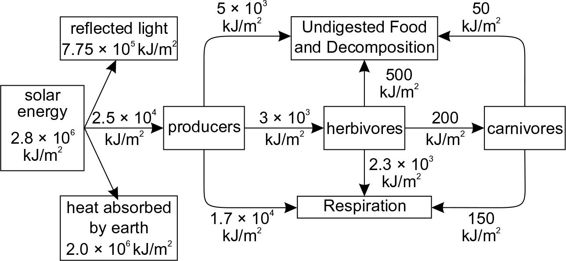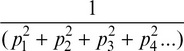# AP Biology Grid-Ins Practice Test 7

### Test Information6 questions9 minutes

1. The yellow color of starch in a corn kernel is determined by a dominant allele that codes for the expression of carotenoid pigments. If no carotenoid pigments are produced, the color is white. The following table provides the number of each type of plant as determined by the farmer after harvesting. Assuming that the crop is in Hardy-Weinberg equilibrium, what is the proportion of plants that are heterozygous for this trait? Provide your answer as a number between 0 and 1 to the nearest hundredth.

Starch Color           Number of Plants

Yellow           1120

White           112

2. The behavior of Anolis lizards is influenced by the altitude of their habitats: the higher the altitude, the greater the amount of time that lizards bask in the sun. The following graph tracks the body temperatures of two species of Anolis at various habitat altitudes. According to the information in the graph, how many degrees warmer is the body temperature of an A. shrevei lizard at 2000 m than that of an A. cybotes lizard at 5 m when the air temperature is 25°C? Express your answer in °C to the nearest tenth.3. Photosynthetic activity can vary with both light intensities and leaf temperature. Solid lines in the following graph plot the rate of photosynthesis as a function of carbon dioxide uptake, leaf temperature, and light intensity. The dashed line plots the rate of respiration that occurs at the same time as photosynthesis. The compensation point occurs when photosynthesis and respiration rates are equal. According to the information in the graph, how much warmer is leaf temperature at the compensation point when light intensity is 11.5 kJ/m2/min than when light intensity is 2.7 kJ/m2/min? Express your answer in °C to the nearest tenth.4. The following figure shows the transfer of energy through a forest community. According to the information in the figure, what is the proportion of energy entering the first trophic level that is passed to the second trophic level? Express your answer as a value between 0 and 1, rounded to the nearest hundredth.5. The following equation describes the hydrolysis of ATP.

ATP + H2O → ADP + Pi

It is estimated that the amount of ATP that is hydrolyzed each day by humans is equivalent to their body weight. If the Gibbs free energy for this reaction is –30 kJ/mole and the molecular weight of ATP is 507 g/mole, how much energy (in kJ) is released by a 50 kg woman each day? Express your answer as a positive whole number.

6. Simpson's index is a method for quantifying the species diversity of a community. The index is determined by the following formula:where each value of p represents the relative abundance of a species. Using the relative abundances given in the following table for a community of six mammal species, calculate Simpson's index. Express your answer to the nearest tenth.

 Species Black Bear Chipmunk Coyote Ground Squirrel Marmot Pika Total Absolute Abundance 2 35 15 20 10 18 100 Relative Abundance 0.02 0.35 0.15 0.20 0.10 0.18 1